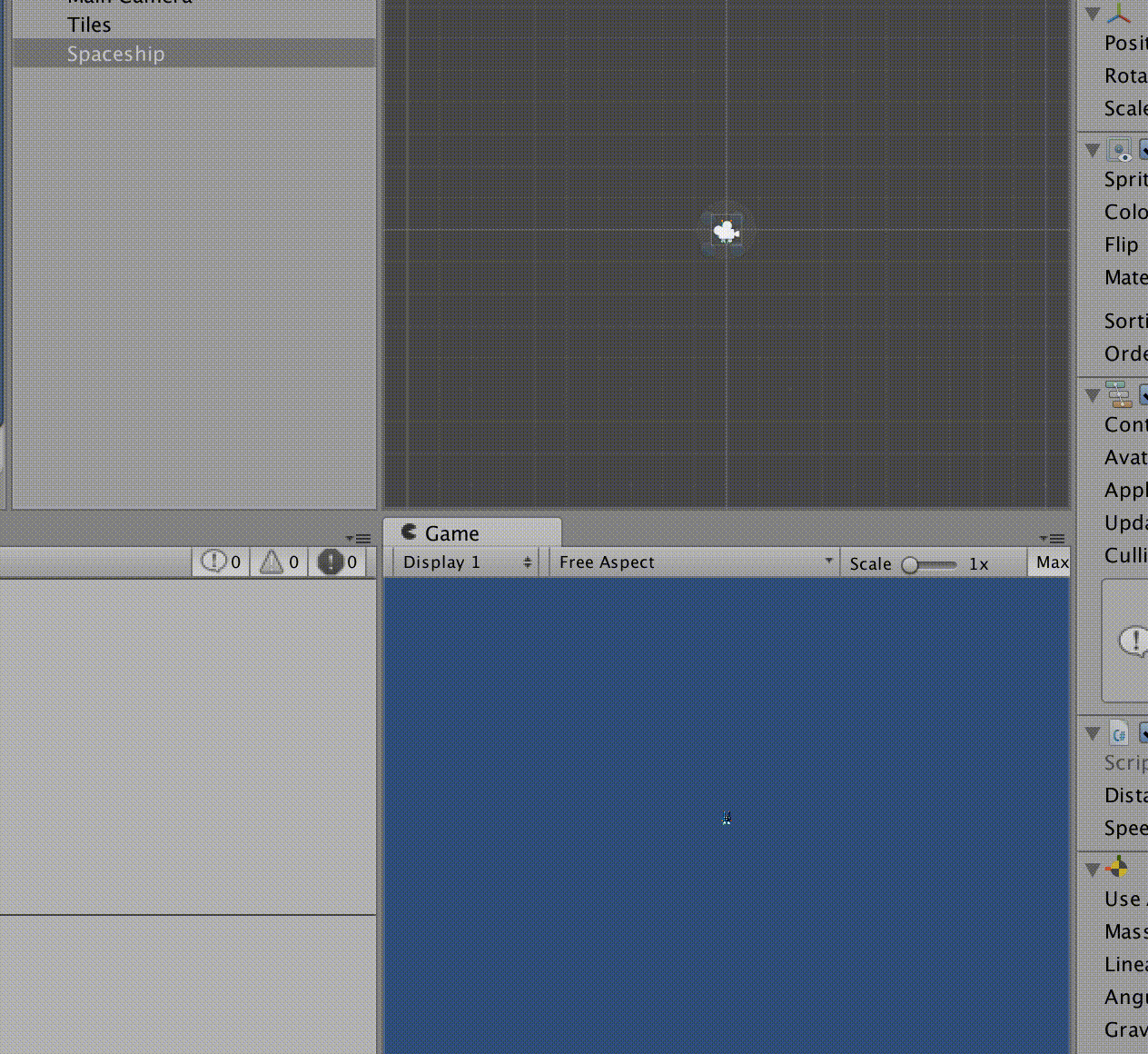I recently I took a shot at implementing an A* (a-star) pathfinding algorithm in Unity. Much of the work draws from Red Blob’s C# implementation of A*, with a few changes to make it work in a Unity project and a little bit of optimization. It seems to be working well, but keep in mind that this is my first attempt and I’m still new to Unity. Also, I don’t expect to keep updating the script I’ve uploaded here as I work on it within my project.

If you’re new to pathfinding (like me), check out Red Blob’s wonderfulpathfinding tutorial for a primer. The script is extensively commented, but here are a few notes:

This `enum` defines the types of possible tiles to `SquareGrid`:

``````public enum TileType {
Floor = 1,
Forest = 5,
Wall = System.Int32.MaxValue
}
``````

Note that Floor, Forest and Wall are somewhat arbitrary, but also represent three different types of tiles, which are all handled differently by A*. Floors are Passable, walls are not, and forests are passable with a higher movement cost. You’ll want to customize this to fit your use case. One thing to consider is to create a type like `Occupied`, so you can keep track of tiles that sprites are currently using.

The `DIRS` array is also worth checking out:

``````public static readonly Location[] DIRS = new [] {
new Location(1, 0), // to right of tile
new Location(0, -1), // below tile
new Location(-1, 0), // to left of tile
new Location(0, 1), // above tile
new Location(1, 1), // diagonal top right
new Location(-1, 1), // diagonal top left
new Location(1, -1), // diagonal bottom right
new Location(-1, -1) // diagonal bottom left
};
``````

The `DIRS` array is important in the pathfinding step where we look for a given tile’s neighbors. In Red Blob’s tutorial and implementation, it only looked for neighbors above, below, and next to each tile. That leads to paths along straight lines and prohibits diagonal movement, which feels weird when you see it in action. So I added diagonals to the directions above. I’ve noticed at least one issue this creates, though. If there are two walls that are diagonal to each other, the sprite can pass diagonally through them. I have a few ideas to solve this, but if anyone knows a good solution, please leave a comment.

For diagonal movement to work well, we also have to make diagonal movement cost a little more than regular movement:

``````// If the heuristic = 2f, the movement is diagonal
public float Cost(Location a, Location b) {
if (AStarSearch.Heuristic(a,b) == 2f) {
return (float)(int)tiles[b.x,b.z] * Mathf.Sqrt(2f);
}
return (float)(int)tiles[b.x,b.z];
}
``````

If the heuristic between a and b is 2, the movement is diagonal and its cost should be the cost of the tile multiplied by the square root of 2. Where each of the sides of a floor tile have a length of 1, for exmple, Sqrt(2) is the literal length of the diagonal. And we can apply the same forumla to tiles of higher costs as well.